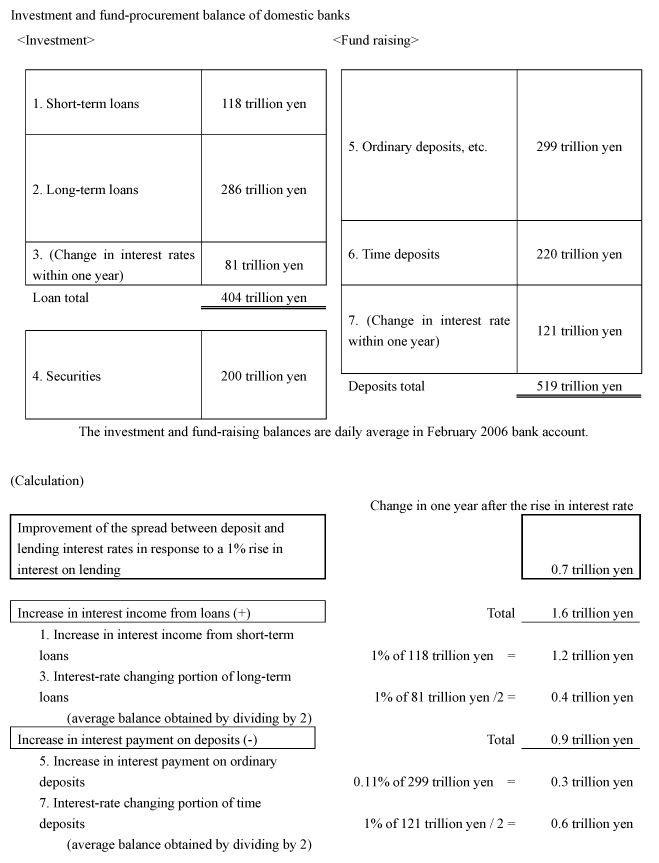Appended Note 1-5 Impact of a Rise in Lending Interest Rates on Banks' Return on Funds(Premise of calculation)Assumed a 1% rise in interest rate on time deposits in response to a 1% rise in lending interest rate
As to interest rate on ordinary deposits, the value of elasticity was calculated by regression by performing a regression of the contractual interest rate and ordinary deposit interest rate (estimation period: 1994-February 2006); Elasticity of interest rate on ordinary deposits = 0.11Long-term loan / short-term loan ratio: The ratio long-term loans with interest-rate changing within one year was calculated from the equations below by using the average contractual interest rate.
Overall average interest rate = Ratio of short-term loans x Short-term loan interest rate + (1 - Ratio of short-term loans) x Long-term loan interest rate
Long-term loan interest rate (stock) = Ratio of interest-rate changing long-term loans (new) x Long-term with interest-rate + (1 - Ratio of interest-rate changing long-term loans) x Long-term loan interest rate (stock in the previous month)
The ratio of long-term loans with interest-rate changing within one year is cumulative of past 12 month figures.
Short-term loans / Total loans = 29.3%
Long-term loans with interest-rate changing within one year / Long-term loans =28.3%Ordinary deposits, etc. = Deposits - Time depositsRatio of time deposits with interest-rate changing within one year:
As to those in deposit less than one year and those with remaining life of one year or more, they are divided by their respective term, and those obtained are treated as deposits with interest-rate changing within one year in the calculation.
Long-term loans with interest-rate changing within one year / Time deposits = 55.2%
Each ratio is the average of past one year.
The calculation above should be approached allowing a certain amount of leeway, because it is based on several assumptions, including interest-rate sensitivity and composition of loan balance. Since a shift of funds from ordinary deposits to time deposits is expected when interest rates are actually raised, the improvement in banks' return on funds may be smaller than the calculation shows.

 Sources: Assets and Liabilities of Domestically Licensed Banks, Average Contracted Interest Rates on Loans and Discounts, and Time Deposits New Receipts and Outstandings, Bank of Japan